1665388500

# Equations.jl: Derive Mathematical Relations

## Equations

Calculate with symbols as numbers:

``````:x+:y
:x*:y
:x/:y
:x^3
sqrt(:x)&@equ x=y^2 # :y
simplify(:a/:a) # 1, so beware of cases where a=0
print(simplify((:a+:b)^2)) # a a + 2 a b + b b
``````

Specify equations conveniently with the equ macros:

``````x=@equ x=a*b^sqrt(y)+c/d
x&@equs(a=3, b=2, y=9, c=8, d=4)
``````

Operate on equations:

``````tri=@equ c^2=a^2+b^2
print(sqrt(tri))
#c = √(a a + b b)
``````

Interpolate with \$:

``````b=3;@equ a=\$b #:a ≖ 3
@equ P=Ten(\$(map(x->pi^x,1:3)),i)
@equs(e=\$ℯ, pi=\$pi, M=\$(rand(3,2)))
``````

Substitute with & (see the plasma tests for real usage examples):

``````energy=@equ E=m*c^2
c=@equ c=299792458
m=@equ m=3*n
n=@equ n=9
@assert (energy&c&m&n).rhs == 3*9*299792458^2
``````

& also does pattern matching:

``````print((Der(:x^:n,:x)-Der(-0.1*:x^:m,:x)+1/:a*Der(:a*sqrt(:x),:x))&relations["Der"])
#n Pow(x,n + (-1)) + 0.1 m Pow(x,m + (-1)) + 0.5 /(Pow(x,0.5))
``````

Write your own patterns as equations:

``````relation=@equ Log(:a,:a)=1
Log(:e)&relation
Log(9,9)&relation
Log(:x+:y,:x+:y)&relation
``````

Use the Oneable type for optional coefficients:

``````rel=@equ Oneable(a)*x*z=y
:q*:r&rel
``````

& is overloaded to apply custom functions enabling chains of arbitrary behavior:

``````eq=3*:x^2-5*:x+1.5 ≖ 0
@assert evaluate(eq.lhs,Dict(:x=>meq.rhs))==0
f1(ex::Expression)=ex
f2(fac::Factor)=3*fac
@assert (:a+:b)&[f1,f2]==3*:a
f3(eq::Equation)=eq'
f4(eq::Equation)=sqrt(eq)
@equ(a=b^2)&[f3,f4]
``````

Tensors are available! The summation convention applies automatically. See the tensors file in examples for usage.

``````Ten(:I,[:i,:i])&@equ I=[1 0;0 1] # 2
Ten(:A,[:i,:i])&@equ A=[:a 0;0 :b] # a+b
Ten(:A,:i)*Ten(:B,:j)&@equs(A=[1,2,3],B=[3,2,1], j=i)
Ten(:A,[:j,:i,:i])*Ten(:B,:j)&@equs(A=ones(3,3,3), B=[1,2,3]) # 18
Alt([:i,:j,:k])*Ten([:a1,:a2,:a3],:j)*Ten([:b1,:b2,:b3],:k)&@equ i=1
``````

To include units use the U type (sensitive to ordering, put unitless stuff last):

``````l=U(:l,:meter);t=U(:t,:second);v=l/t;print(v)
``````

Equations can also be constructed without macros (the ≖ is written as \eqcirc+tab) and results derived by checking for matches:

``````rule=Der(:a*:x,:x)≖:a # equivalent to Equation(Der(:a*:x,:x),:a)
ex=Der(3*:x,:x)
m=matches(ex,rule) # equivalent to ex&rule
``````

Simplification is automatic when using & however sometimes has to be carried out manually:

``````simplify((:x+:y)^3)
simplify(:x*:y/:x)
simplify(sqrt(:x*:z*:y*:z*:y*:x))
``````

Equations have a left hand side (lhs) and a right hand side (rhs) that when omitted defaults to 0.

``````eq=Equation(:x*:z+:y)
eq.rhs
matches(eq)
eq=Equation(:x^2,9)
matches(eq,Sqrt)
``````

If you try to evaluate an equation that has been constructed through division matching by setting one of the divided symbols to zero an error will be thrown:

``````meq=matches(:x^2+:a*:x≖0,Div)
evaluate(meq,Dict(:x=>0))``````

Author: jhlq
Source Code: https://github.com/jhlq/Equations.jl

## Buddha Community1617959650

## A reliable Cryptocurrency Derivatives Exchange Development company

Antier Solutions is consider as one of the best Cryptocurrency Derivatives Exchange Development company that is specialized in offering world’s best cryptocurrency Derivatives Exchange Development Services that enables the following 4 key types- Futures trading, Forwards trading, Options trading, and Perpetual trading. Our skilled team of blockchain developers build Derivative exchange on both centralized and decentralized platform. It’s your choice to build the best one for you. Exchange owners can leverage the potential of a cryptocurrency derivatives exchange to reach more investors. A Crypto derivative trading platform is more flexible than Spot Margin trading and opens access to otherwise unavailable markets. To know more about us, feel free to visit us.

#cryptocurrency derivatives exchange #crypto derivatives exchange development #cryptocurrency derivatives exchange development #derivatives exchange development services #derivative exchange development company1597089600

## Using Mathematical Modeling to Simulate an Epidemic

In this article, we’ll explore and visualize a classic mathematical model used for modeling the spread of infectious disease: the SIR model.

### Population compartments

The model splits the population into three compartments:

• The susceptible (S): Individuals who are yet to contract the disease.
• The** infectious** (I): Individuals who have contracted the disease and are capable of spreading it to susceptible individuals.
• The **recovered **®: Individuals who have recovered from the disease and became immune to it.

The sum of these compartments represents the number of individuals in the population, which is denoted by N: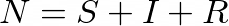Our goal is to model how these compartments fluctuate over time, and so we’ll consider them to be functions with respect to time:The SIRD model considers another compartment (D) for deceasedindividuals.

### Compartment transition

In the SIR model, the transition between compartments takes the following path: susceptible → infectious → recovered. Each transition happens at a different rate.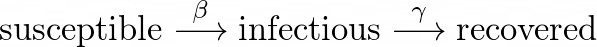The rate at which susceptible individuals come into contact with infectious individuals, thus contracting the disease, is called the **infectious rate **(β). Once infected, the rate at which they recover is called the **recovery rate **(γ).

The SIRS model takes into account the possibility of recovered individuals becoming susceptible again, due to loss of immunity.

## The model equations

To model the fluctuation of the population compartments, we need to derive formulas to express the way they change with respect to time.

### The susceptible equation

Let’s consider an initial moment _tₒ. _Each day, an infectious individual spreads the disease to a fraction of the susceptible individuals, which become infectious themselves.At some moment t, the number of new infectious individuals is:And so, by subtracting the new infectious individuals from the initially susceptible ones, we obtain:To get the instantaneous rate of change, we divide by (_t- tₒ) _and take the limit as t approaches tₒ:This limit is nothing but the derivative of S with respect to time at some point tₒ. And so:### The infectious equation

Again, let’s consider an initial moment _tₒ. _Each day, new individuals become infectious, and some of the already infectious recover.

We saw previously that:Furthermore,By combining these observations, we can write:To get the derivative of I, by dividing by (_t- tₒ) _and taking the limit as _t _→ _tₒ, _we get:which means that:### The recovered equation

Same as before, let’s consider an initial moment _tₒ. _Each day, a part of the infectious individuals recover.

We saw that:And so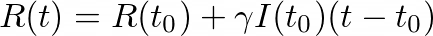As we did previously, we’ll divide by (_t- tₒ) _and take the limit as _t _→ _tₒ _to get the derivative of R:### Unifying the model

Putting the three formulas together gives us the SIR model system of ODEs:Or, by lettingthe system becomes:Put in this form, we can simply treat the system as an ODE.

## Visualizing the model

We can observe that this system above is non-linear (analytic solutions can, however, be found in an implicit form). Nonetheless, since our purpose is to visualize the model, we’ll resort to a numerical method to solve the system.

### The Euler method

The Euler method is a procedure for solving ordinary differential equations numerically given an initial value.

For our case, we’ll consider the number of individuals in each compartment to be known at tₒ = 0:The Euler method consists of iterating through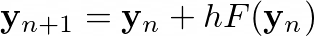where h is the chosen step. By performing this, we can approximate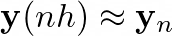### Coding the solution

Let’s write some code to find and plot the solution:

``````	import numpy as np
import matplotlib.pyplot as plt
from matplotlib.animation import FuncAnimation

class SIR:
def __init__(self, beta, gamma, S0, I0, R0):
self.beta = beta
self.gamma = gamma

self.y0 = np.array([S0, I0, R0])
self.N = sum(self.y0)

def F(self, y):
S, I, R = y
return np.array([ -self.beta * I * S / self.N, self.beta * I * S / self.N - self.gamma * I, self.gamma * I])

def euler(self, tmax, h):
y0 = self.y0
t = 0

while t <= tmax:
y = y0 + h * self.F(y0)
yield t, y

y0 = y
t += h

def plot(self, days):
X, Y = [], []
h = .2

for t, y in self.euler(days, h):
X.append(t)
Y.append(y)

fig, ax = plt.subplots()
ax.set_xlim(0, days)
ax.set_ylim(0, self.N)

S_line, = ax.plot(0, 0, color='#4FA1E4')
I_line, = ax.plot(0, 0, color='#FE2020')
R_line, = ax.plot(0, 0, color='#40D752')

def iter(i):
S_line.set_xdata(X[:i])
I_line.set_xdata(X[:i])
R_line.set_xdata(X[:i])

Yt = np.transpose(Y[:i])

S_line.set_ydata(Yt)
I_line.set_ydata(Yt)
R_line.set_ydata(Yt)

return S_line, I_line, R_line,

anim = FuncAnimation(fig, func=iter, frames=range(1, int(days/h)), interval=50, repeat=False)

plt.xlabel('Day')
plt.ylabel('Population')
plt.legend(['Susceptible', 'Infected', 'Recovered'], frameon=False, loc='upper center', ncol=3)
plt.title('SIR model')

plt.show()
``````

#simulation #mathematical-modeling #visualization #mathematics #differential-equations1665388500

## Equations

Calculate with symbols as numbers:

``````:x+:y
:x*:y
:x/:y
:x^3
sqrt(:x)&@equ x=y^2 # :y
simplify(:a/:a) # 1, so beware of cases where a=0
print(simplify((:a+:b)^2)) # a a + 2 a b + b b
``````

Specify equations conveniently with the equ macros:

``````x=@equ x=a*b^sqrt(y)+c/d
x&@equs(a=3, b=2, y=9, c=8, d=4)
``````

Operate on equations:

``````tri=@equ c^2=a^2+b^2
print(sqrt(tri))
#c = √(a a + b b)
``````

Interpolate with \$:

``````b=3;@equ a=\$b #:a ≖ 3
@equ P=Ten(\$(map(x->pi^x,1:3)),i)
@equs(e=\$ℯ, pi=\$pi, M=\$(rand(3,2)))
``````

Substitute with & (see the plasma tests for real usage examples):

``````energy=@equ E=m*c^2
c=@equ c=299792458
m=@equ m=3*n
n=@equ n=9
@assert (energy&c&m&n).rhs == 3*9*299792458^2
``````

& also does pattern matching:

``````print((Der(:x^:n,:x)-Der(-0.1*:x^:m,:x)+1/:a*Der(:a*sqrt(:x),:x))&relations["Der"])
#n Pow(x,n + (-1)) + 0.1 m Pow(x,m + (-1)) + 0.5 /(Pow(x,0.5))
``````

Write your own patterns as equations:

``````relation=@equ Log(:a,:a)=1
Log(:e)&relation
Log(9,9)&relation
Log(:x+:y,:x+:y)&relation
``````

Use the Oneable type for optional coefficients:

``````rel=@equ Oneable(a)*x*z=y
:q*:r&rel
``````

& is overloaded to apply custom functions enabling chains of arbitrary behavior:

``````eq=3*:x^2-5*:x+1.5 ≖ 0
@assert evaluate(eq.lhs,Dict(:x=>meq.rhs))==0
f1(ex::Expression)=ex
f2(fac::Factor)=3*fac
@assert (:a+:b)&[f1,f2]==3*:a
f3(eq::Equation)=eq'
f4(eq::Equation)=sqrt(eq)
@equ(a=b^2)&[f3,f4]
``````

Tensors are available! The summation convention applies automatically. See the tensors file in examples for usage.

``````Ten(:I,[:i,:i])&@equ I=[1 0;0 1] # 2
Ten(:A,[:i,:i])&@equ A=[:a 0;0 :b] # a+b
Ten(:A,:i)*Ten(:B,:j)&@equs(A=[1,2,3],B=[3,2,1], j=i)
Ten(:A,[:j,:i,:i])*Ten(:B,:j)&@equs(A=ones(3,3,3), B=[1,2,3]) # 18
Alt([:i,:j,:k])*Ten([:a1,:a2,:a3],:j)*Ten([:b1,:b2,:b3],:k)&@equ i=1
``````

To include units use the U type (sensitive to ordering, put unitless stuff last):

``````l=U(:l,:meter);t=U(:t,:second);v=l/t;print(v)
``````

Equations can also be constructed without macros (the ≖ is written as \eqcirc+tab) and results derived by checking for matches:

``````rule=Der(:a*:x,:x)≖:a # equivalent to Equation(Der(:a*:x,:x),:a)
ex=Der(3*:x,:x)
m=matches(ex,rule) # equivalent to ex&rule
``````

Simplification is automatic when using & however sometimes has to be carried out manually:

``````simplify((:x+:y)^3)
simplify(:x*:y/:x)
simplify(sqrt(:x*:z*:y*:z*:y*:x))
``````

Equations have a left hand side (lhs) and a right hand side (rhs) that when omitted defaults to 0.

``````eq=Equation(:x*:z+:y)
eq.rhs
matches(eq)
eq=Equation(:x^2,9)
matches(eq,Sqrt)
``````

If you try to evaluate an equation that has been constructed through division matching by setting one of the divided symbols to zero an error will be thrown:

``````meq=matches(:x^2+:a*:x≖0,Div)
evaluate(meq,Dict(:x=>0))``````

Author: jhlq
Source Code: https://github.com/jhlq/Equations.jl1597913100

## To Relational or Not to Relational?

Working on your next project but not sure which back-end technology to use? For starters, databases come in two flavors: relational and non-relational. Both have their own set of rules, pros and cons which help in determining which type of application they should be used in. Let us delve into these differences so that we would be able to decide which one is better suited for us.

## Relational Databases:

Relational databases store data in the form of tables containing rows (representing entries) and columns (containing a set of information) having a unique key for each row. These keys can either be primary or foreign. It is based on a form of algebraic set theory known as relational algebra, which is used in the relational modeling of data. They are better suited for applications where many transactions are involved, also known as transactions-oriented applications (OLTP).

They use SQL (Structured Query Language) as a language for the maintenance and querying of databases. We can use MySQL, SQL Server, SQLite, MS Access, Oracle, Sybase, PostGreSQL, or Informix.

They are normalized databases i.e they are in accordance with a series of so-called “normal forms” in order to reduce data redundancy and improve data integrity.

1. They are useful for applications requiring a lot of transactions, complex queries and routine analysis of data

2. They are robust, simple, structured and flexible.

3. They ensure reliable data transactions through ACID (Atomity, Consistency, Isolation, Durability).

4. Easier to use because of GUI (Graphical User Interface)

1. Their main disadvantage is that it is impossible to horizontally scale them. If our business requirements increase, then we need to move on to bigger systems, making them more costly in the long run.

2. Images and other multimedia files cannot be stored.

3. We cannot use them for geospatial data

4. They can cause ORM Impedance Mismatch

Here_ is a basic CRUD demo of __MySQL _using Node.js. You need to install the relevant software before running the examples in the repository.

#non-relational-database #mysql #relational-databases #nodejs #mongodb1596998880

## Everything You Always Wanted to Know About Derivatives

You’re sitting in a classroom. You look around and see your friends writing something down. It seems they are taking the exam, and they know all the answers (even Johnny who, how to say it… wasn’t the brilliant one). You realize that your exam is in front of you, and it’s Maths. You start reading it but you don’t understand a thing. That’s terrible, your heart speeds up, you’re sweating and then… you wake up.

Uff, it was only a dream. You get back to sleep, but one thing bothers you. This paper from a dream, there was something about… how is it called… derivatives? You remember you learned it by heart at school, but never truly understood it. It’s time to face this ghost from the past.

## Don’t be afraid, it’s there for a while

The idea of the derivative is not new. There is a little bit of controversy about the inventor of derivatives. The battle is between Sir Isaac Newton and Gottfried Wilhelm Leibniz.

Apparently, these two great minds discovered it independently, not being aware of their colleagues’ work. What’s interesting they came to similar conclusions having completely different ideas and approaches to the problems they were trying to solve.

Newton thought in the context of physics and motion, while Leibniz thought in terms of formula that could describe a change in the metaphysical meaning. However, people worked on similar theorems before the 17th century, when Newton & Leibniz lived. Arab and Persian mathematicians from the 11th and 12th centuries are supposed to discover basic ideas behind derivatives.

## And it’s useful!If you have ever been wondering if you’ll use any of the things you learned at school in real life, the answer is yes, derivatives are such a thing. Nowadays they are an important part of algorithms in many innovative areas, like Artificial Intelligence. In Machine Learning which is one of the AI domains, derivatives help computer programs to learn.

Generally speaking, such algorithms optimize objective functions (very often derivatives are needed for this purpose) so programs can find optimal parameters to solve different tasks (e.g. recognizing people on photos). Let’s get the key idea behind derivatives.

#derivatives #math #data-science #learning #mathematics #deep learning